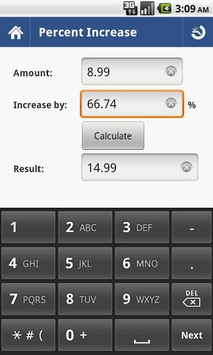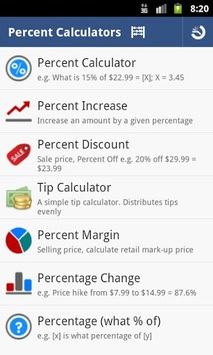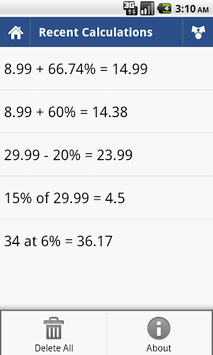# Percent Calculator APK

Simplest Percent Calculator. Enter any two values to compute the third.

### Screenshots## Percent Calculator APK Description

1. Calculate Percents & Percentages
2. Percent Discounts (sale price)
3. Percent Markups (increase by)
4. Percent Margin (selling price)
5. Calculate Tips.
6. Percentage Difference (Change)
7. Percentage (what % of) i.e. x is what percentage of y

Tip:
Enter any two values and the third is computed e.g. % of [x] = 115. X will be computed (20).
Customize background colors.